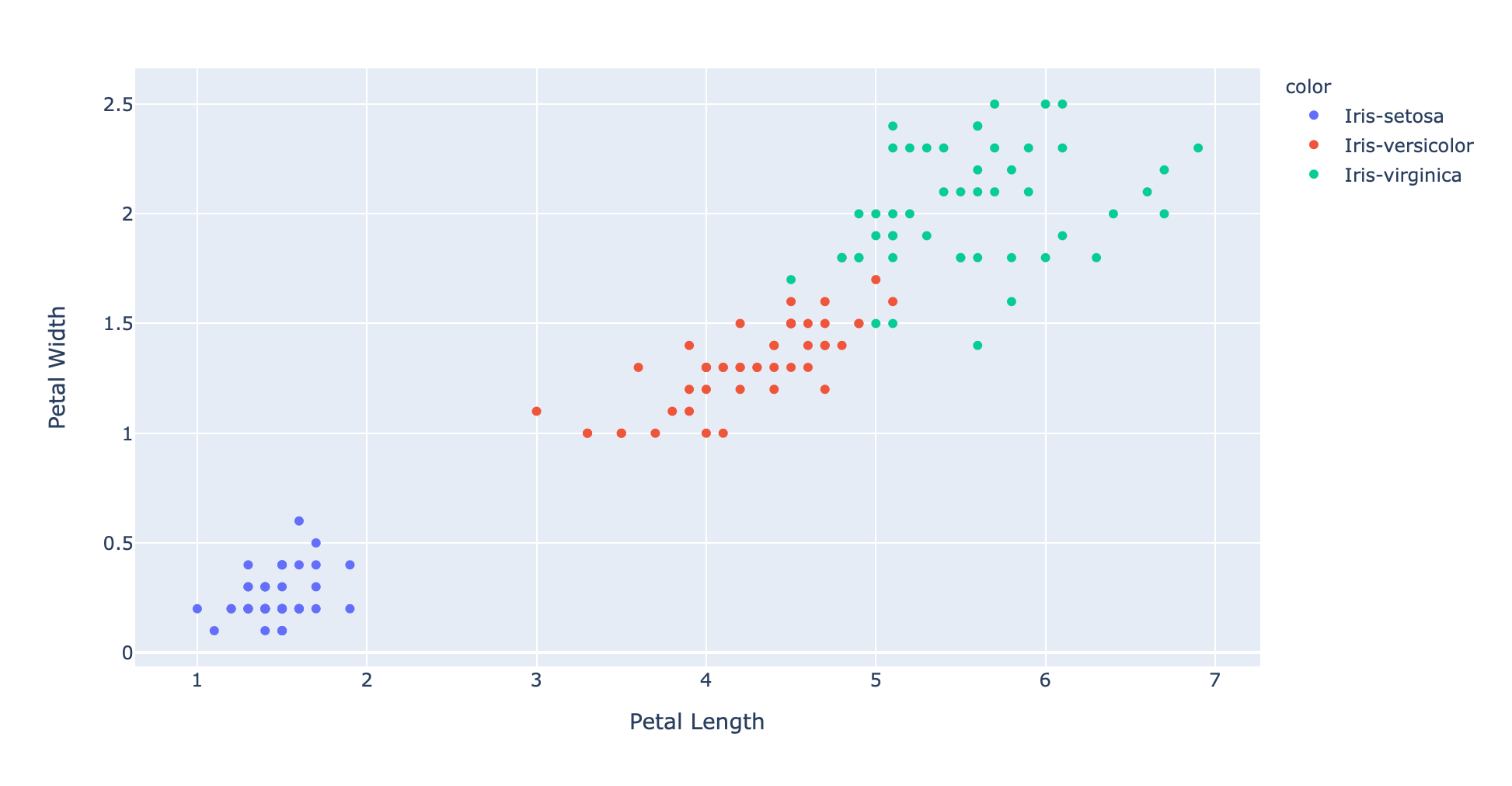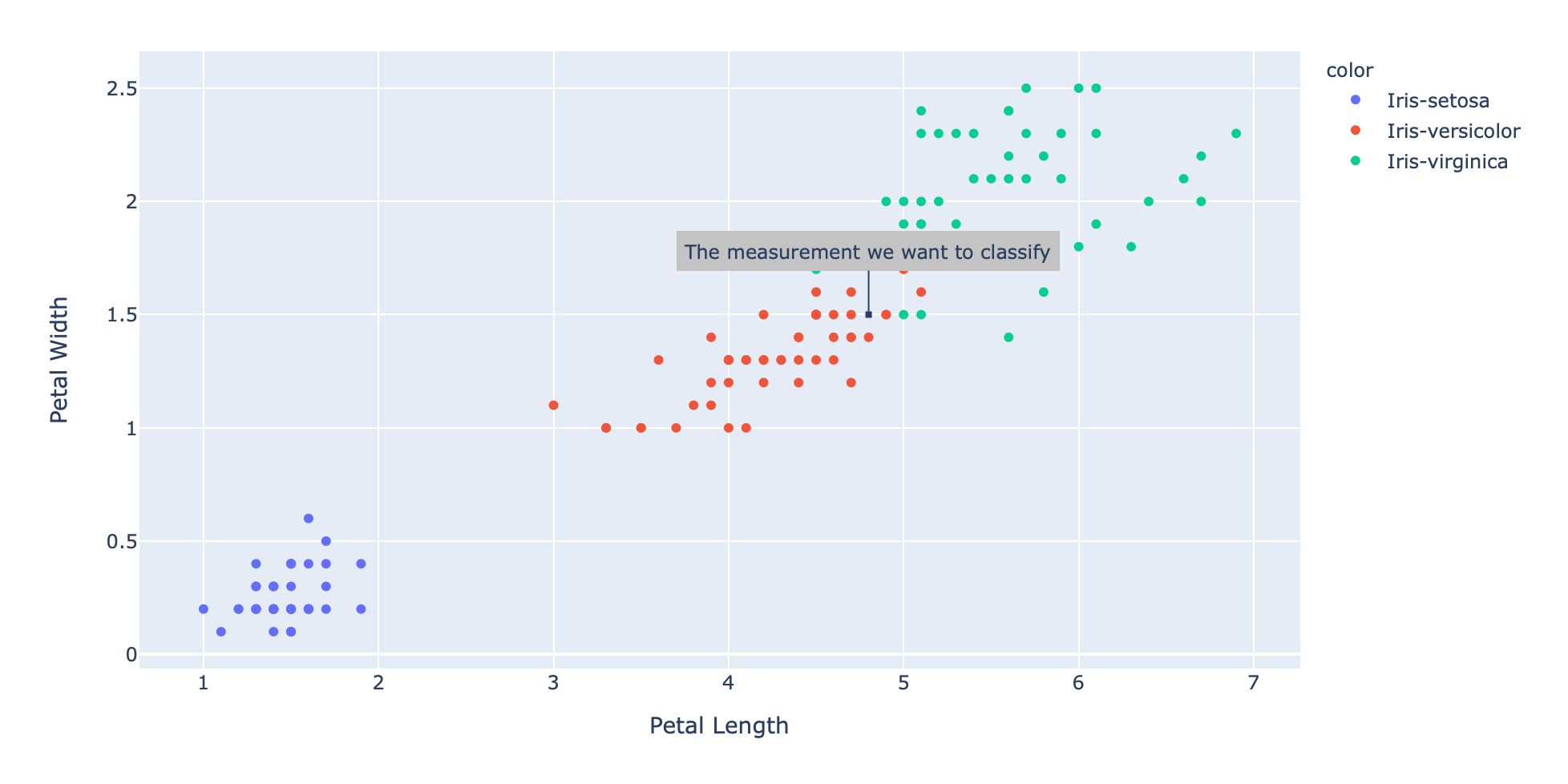## 写给小白：K近邻算法入门K近邻（简称K-NN或KNN）是一种简单而优雅的机器学习算法，用于根据现有数据对不可见的数据进行分类。该算法的优点是不需要传统的训练阶段。如果存在分类问题和标记数据，则可以利用现有的已分类数据，预测任何不可见的数据类别。

## 原理

• weight: 重量（千克）
• height: 高度（厘米）

$$\begin{bmatrix}0.5\0.8\0.1\end{bmatrix}=Podenco$$

## 两个概念

### 距离函数

$$d(x, y)=d(y, x)=\sqrt{\sum_{i=1}^N(x_i-y_i)^2}$$

$$\overrightarrow{a}=\begin{pmatrix}1\2\3\4\end{pmatrix} \overrightarrow{b}=\begin{pmatrix}5\6\7\8\end{pmatrix}$$

$$d(\overrightarrow{a},\overrightarrow{b})=d(\overrightarrow{b},\overrightarrow{a})=\sqrt{(1-5)^2+(2-6)^2+(3-7)^2+(4-8)^2}$$$$=\sqrt{64}=8$$

## K-NN算法

knn算法的首要任务是计算新数据点和所有其他现有数据点之间的距离。之后，我们需要从最近到最远的距离排序，并提取数据点标签。然后截断此有序列表，使其仅包含k个最近的数据点标签。最后一步是将此列表传递给投票函数，该函数用于计算预测标签。

## 鸢尾花分类

• Iris Setosa
• Iris Virginica
• Iris Versicolor## 结论

k近邻分类算法是一种非常强大的分类算法，它可以根据已有标签的数据来标记缺失标签的数据。k-NNs的主要思想是：利用新的“待分类”数据点的K个最近邻来“投票”选出它应有的标签。